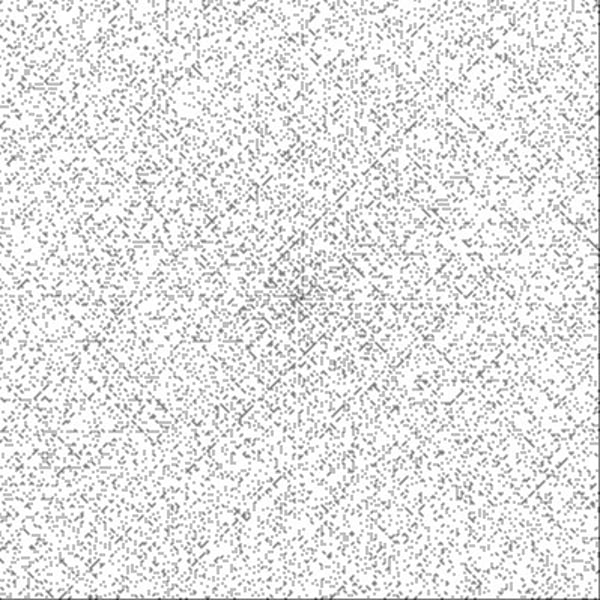Related Tags

# What are spiral primes?

Samia Ishaque

Spiral primes, also known as Ulam’s primes, are a way of arranging prime numbers to see the patterns they form. Stanislaw Ulam discovered spiral primes in 1963 by arranging positive integers in a spiral and then circling all the prime numbers along the spiral. The patterns formed by this were shocking, as the primes mostly fell along diagonal lines. This discovery was later published in the Scientific Discovery magazine in 1964.

Below is a picture of a 399 by 399 grid of numbers, where the prime numbers have all been dotted:## Explanation

The patterns in the number spiral follow the equation:

$f(n)=4n^{2} + bn + c$

where $b$ and $c$ are integers. The values of $b$ can decide the shape of the pattern. If $b$ is even, diagonals are formed. This equation also explains why some numbers have a higher concentration of prime numbers than others. For example, numbers lying along one polynomial equation may be more divisible than another polynomial.

RELATED TAGS

CONTRIBUTOR

Samia Ishaque